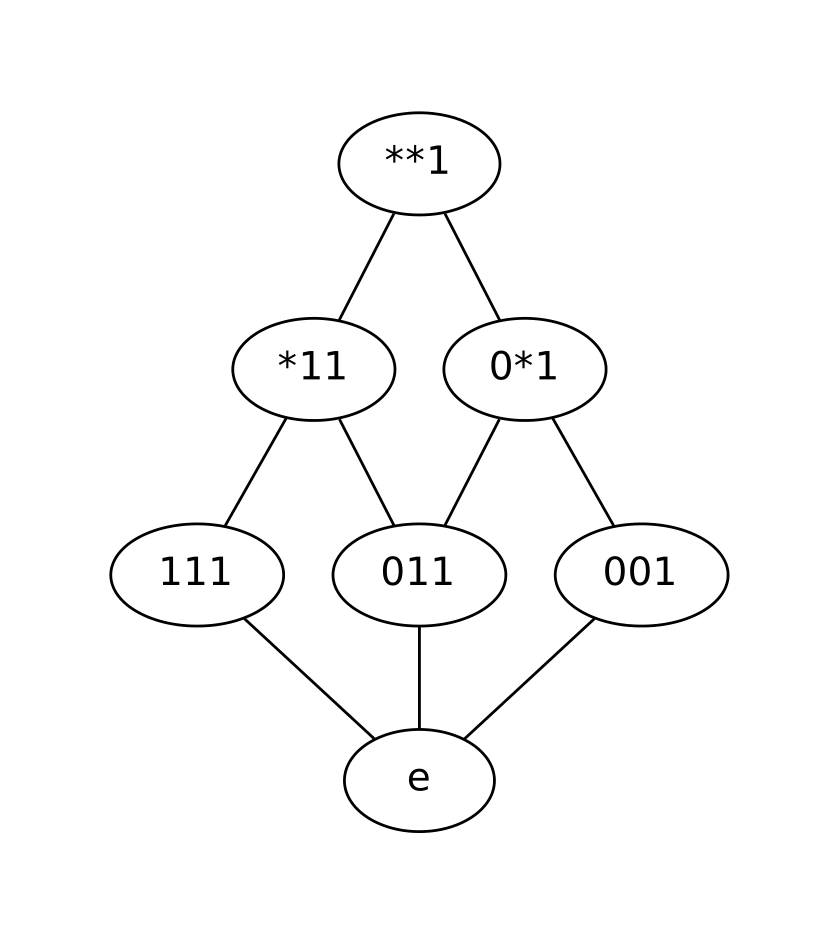A python package for all things schemata related

## Project description

schematax is a simple Python package to do all things related to schemata.

A schema is word made using an extra symbol, ‘*’, called the wild card symbol. For example the schema over the binary aplhapbet ‘1*0’, represents the set of strings. {‘100’,’110’}’.

Schema have properties. For example, for a schema s, the order of s is the number of symbols in s which are not the wild card symbols (called fixed symbols). The defining length of s is the distance between the first and last fixed symbol.

Given a set of words of the same length S, the schematic completion of S returns all schema which make subsets of the words in S. Whats more given the partial ordering over schemata, the schematic completion of S forms a Complete Lattice.

## Installation

This package runs under Python 2.7, use pip to install:

\$ pip install schematax

This will also install the graphviz package from PyPI as required dependencies.

Important: Drawing the the schematic lattice uses Graphviz software. Make sure it is installed and dot executable is on your systems’ path.

## Quickstart

The file example.py gives a good overview of how to use the package.

Basic schema operations:

>>> import schematax

>>> s = schematax.schema('10*1') #makes a schema

>>> s
10*1

>>> s.get_order()
3

>>> s.get_defining_length()
3

>>> s2 = schematax.schema('1**1') #makes another schema

>>> s <= s2
True

>>> s < s2
True

>>> s == s2
False

>>> schematax.meet(s,s2)
10*1

>>> schematax.join(s,s2)
1**1

>>> s3 = schematax.schema('00*1')

>>> schematax.supremum([s,s2,s3])
***1

>>> schematax.infimum([s,s2,s3])
e                               #e stands for the empty schema

Schematic completion and drawing the schematic lattice:

>>> import schematax

>>> xs = ['111', '011', '001']

>>> ss = schematax.complete(xs) #performing schematic completion

>>> ss
[111, 011, 001, *11, **1, 0*1, e] #e stands for the empty schema

>>> schematax.draw(ss,'my_lattice') #draws the schematic lattice of ss and saves it as my_lattice.pdf

The image produced here:The implementation is based on these Python packages:

• graphviz – Simple Python interface for Graphviz

## Project details

This version0.1.6

Uploaded source